### Ordinary Kriging

Denote the SRF by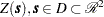. Following the notation in Cressie (1993), the following model for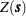is assumed: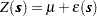Here,is the fixed, unknown mean of the process, and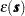is a zero mean SRF, which represents the variation around the mean.

In most practical applications, an additional assumption is required in order to estimate the covariance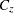of theprocess. This assumption is second-order stationarity: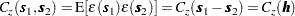This requirement can be relaxed slightly when you are using the semivariogram instead of the covariance. In this case, second-order stationarity is required of the differences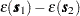rather than: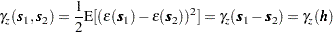By performing local kriging, the spatial processes represented by the previous equation forare more general than they appear. In local kriging, at an unsampled location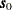, a separate model is fit using only data in a neighborhood of. This has the effect of fitting a separate meanat each point, and it is similar to the kriging with trend (KT) method discussed in Journel and Rossi (1989).

Given the
measurements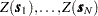at known locations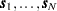, you want to obtain a prediction of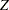at an unsampled location. When the following three requirements are imposed on the predictor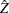, the OK predictor is obtained:

1.is linear in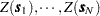2.is unbiased

3.minimizes the mean square prediction error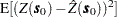Linearity requires the following form for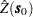: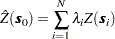Applying the unbiasedness condition to the preceding equation yields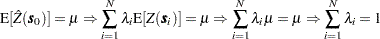Finally, the third condition requires a constrained linear optimization that involves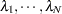and a Lagrange parameter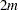. This constrained linear optimization can be expressed in terms of the function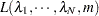given by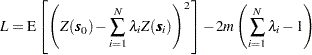Define the
column vector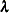by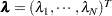and the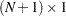column vector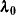by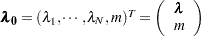The optimization is performed by solving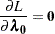in terms of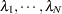and.

The resulting matrix equation can be expressed in terms of either the covariance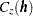or semivariogram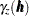. In terms of the covariance, the preceding equation results in the matrix equation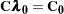where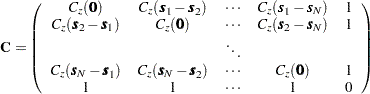and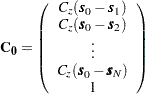The solution to the previous matrix equation is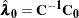Using this solution forand, the ordinary kriging prediction at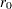is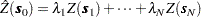with associated prediction error the square root of the variance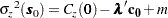where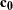is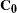with thein the last row removed, making it anvector.

These formulas are used in the best linear unbiased prediction (BLUP) of random variables (Robinson 1991). Further details are provided in Cressie (1993, pp. 119–123).

Because of possible numeric problems when solving the previous matrix equation, Deutsch and Journel (1992) suggest replacing the last row and column ofs in the preceding matrix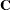by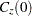, keeping thein the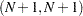position and similarly replacing the last element in the preceding right-hand vectorwith. This results in an equivalent system but avoids numeric problems whenis large or small relative to.